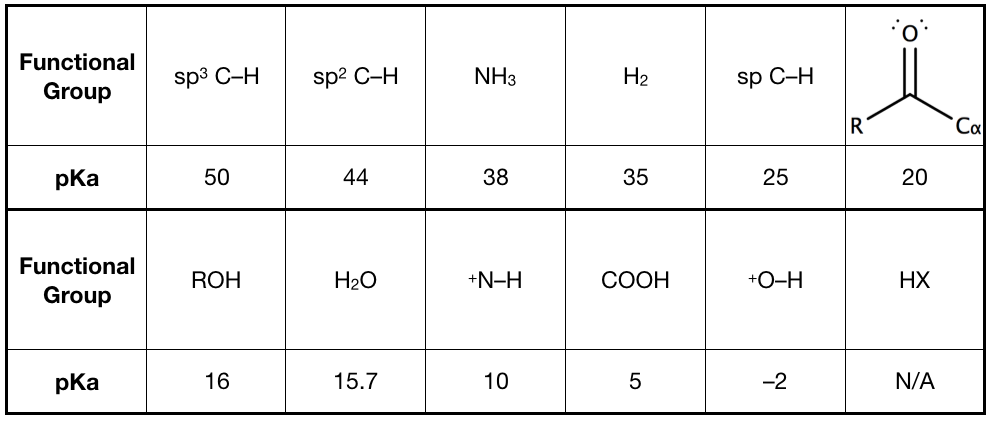# Problem: (a) Arrange the following compounds in order of decreasing acidity and explain your answer: CH3CH2NH2, CH3CH2OH, and CH3CH2CH3.

###### FREE Expert Solution
94% (499 ratings)
###### FREE Expert Solution

We’re being asked to rank the given compounds in order of decreasing acidity.

Recall that pKa is the measure of the acidity of a compound: lower pKa  more acidic.

This means, we simply need to determine the functional group of each compound and the corresponding pKa values. The pKa values are:94% (499 ratings)###### Problem Details

(a) Arrange the following compounds in order of decreasing acidity and explain your answer: CH3CH2NH2, CH3CH2OH, and CH3CH2CH3.

What scientific concept do you need to know in order to solve this problem?

Our tutors have indicated that to solve this problem you will need to apply the pKa concept. You can view video lessons to learn pKa. Or if you need more pKa practice, you can also practice pKa practice problems.

What is the difficulty of this problem?

Our tutors rated the difficulty of(a) Arrange the following compounds in order of decreasing a...as low difficulty.

How long does this problem take to solve?

Our expert Organic tutor, Jonathan took undefined 56 seconds to solve this problem. You can follow their steps in the video explanation above.

What professor is this problem relevant for?

Based on our data, we think this problem is relevant for Professor Myers' class at HARVARD.

What textbook is this problem found in?

Our data indicates that this problem or a close variation was asked in Organic Chemistry - Solomons 10th Edition. You can also practice Organic Chemistry - Solomons 10th Edition practice problems.